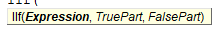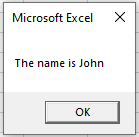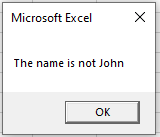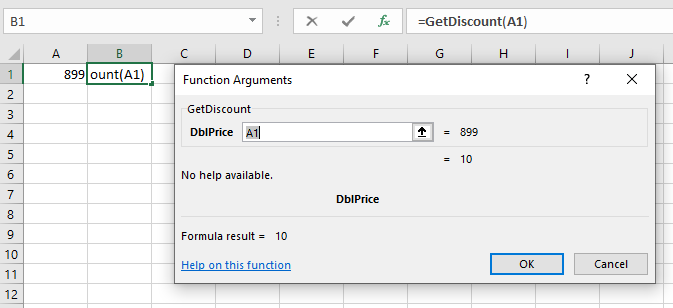# VBA IIF Function

This tutorial will explain the how to use IIF in VBA

The VBA IIF function is similar to using the IF Function in Excel. It tests if a condition is met, returning one value (or calculation) if TRUE, another value (or calculation) if FALSE.

It is similar to the VBA If Statement, many consider it a shortcut to using this method as you only need to write one line of code to get the desired result rather than using an If..Then..Else..End If routine. However, it needs to be used correctly as it can lead to problems in your code.

## IIF Syntax

The IFF function is made up of 3 parts – the logical test, the true part and the false part.• Expression: The logical test that is to take place.
• True Part: The result that will be returned if the logical test is TRUE.
• False Part: The result that will be returned if the logical test is FALSE.

## Writing an IIF Function Procedure

``````Function GetNames(strName As String) As String
GetNames = IIf(strName = "John", "The name is John", "The name is not John")
End Function``````

In the function above, we can use a sub-procedure to test to see if the variable we pass to the function is the string “John”

``````Sub TestGetNamaes()
MsgBox GetNames("John")
End Sub``````

If we were to run the TestGetNames sub-procedure, it would call the GetNames Function and return a message box.If we had used the If method instead, the code would have looked like this:

``````Function GetNames(strName As String) As String
If(strName = "John") Then
GetNames = "The name is John"
Else
GetNames = "The name is not John"
End If
End Function``````

We have effectively written one line of code instead of 5 lines of code –  impressive!

Consider the following

``````Function GetNames(strName As String) As String
GetNames = IIf(strName = "John", MsgBox("The name is John"), MsgBox("The name is not John"))
End Function``````

Now if you run the following Sub-Procedure to call your function

``````Sub TestGetNames()
GetNames ("John")
End Sub``````

You would get the same message box as before, but then immediately after that – you would get the next message box!The IIF function executes both the TRUE and the FALSE sections of the line of code – it does not exit the code once it has found the condition to be true – it still executes the false section as well – thus giving us a false message in the second message box.  If you had used  If..Then..Else..End If – this would not have occurred – the IF function only executes either the TRUE or the FALSE section of the code – depending on the logic being passed to the code.

The code is badly designed (on purpose!) with the message boxes being held within the line of code that the IIF statement is on rather than after the code, or  in the sub-routine.  As the IIF function runs both the TRUE and the FALSE sections of the statement, both messages are returned.

We could fix this error by moving the message box to below the IIF function line, as in the code below, or by moving the message box  to the Sub-Procedure as per the first example in this article.

``````Function GetNames(strName As String) As String
GetNames = IIf(strName = "John", "The name is John", "The name is not John")
MsgBox (GetNames)
End Function``````

If you are careful when you write you code, the IIF function can save you plenty of lines of code and extra typing!

## Nested IIF’s

We can nest the IIF function in a similar fashion to nesting the IF function but once again, everything is done in one line.

``````Function GetDiscount(dblPrice As Double) As Double
GetDiscount = IIf(dblPrice >= 500, 10, IIf(dblPrice >= 250, 5, IIf(dblPrice >= 100, 2.5, 0)))
End Function``````

We could then call this function from a Sub-Procedure

``````Sub FindDiscount()
Dim dblP As Double
dblP = 899
MsgBox ("The discount you can obtain is " & GetDiscount(dblP) & "%")
End Sub``````

or you could call it from within Excel, using it as a UDF (User Defined Function)Stop searching for VBA code online. Learn more about AutoMacro - A VBA Code Builder that allows beginners to code procedures from scratch with minimal coding knowledge and with many time-saving features for all users!